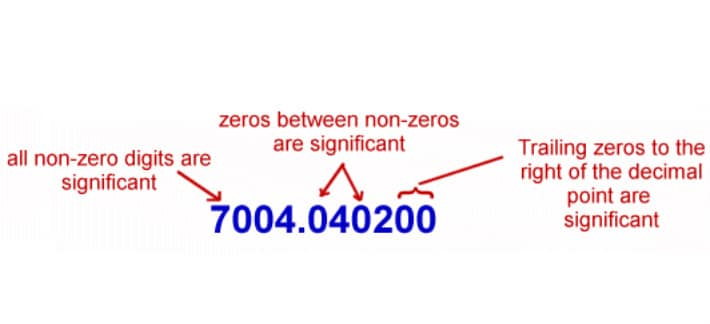• Hire UsUh Oh! It seems you’re using an Ad blocker!

We always struggled to serve you with the best online calculations, thus, there's a humble request to either disable the AD blocker or go with premium plans to use the AD-Free version for calculators.

Or

Blog / Education / 6 Rules Of Significant Figures# 6 Rules Of Significant Figures

No doubt, the appropriate number of significant figures is essential in order to have a meaningful level of resolving power while reporting analytical concentrations. In fact, there are several criteria and methods can be accounts while estimating how many significant figures are needed. Several significant figures rules are considered while determining sig figs of a number. Also, people use a simple sig fig calculator to know how many sig figs an expression has!

## What Experts Says About Sig Figs:

When you take a measurement, you have to write down the correct number of digits, means you ought to write down the significant digits. Remember that the way you write a number indicate some information about how accurate it is. So, enter the number into sig fig calculator to get the significant figures for a given number.

## What is the purpose of significant figures?

The most common purpose of using significant figures is to keep track of the quality (variability) of measurements. That means, propagating that information during the calculations using the measurements.

## Overview of key Factors:

We will emphasize the following points as being most important:

• Significant figures relate to measurements. When you think about how many sig figs a number has, you ought to think about where the number came from
• The crucial rule for handling sig figs when doing calculations is the rule for multiplication

## 6 Rules of Significant Figures:

Rule #1:

Every non-zero digit in a reported measurement is said to be sig figs

Rule #2:

Zeros appearing between non-zero digits are said to be sig figs

Rule #3:

Leftmost zeros appearing in front of non-zero digits are not said to be significant.

Rule #4:

Zeros at the end of a number and even to the right of a decimal point are always said to be significant

Rule #5:

The zeros at the rightmost end of a measurement that lie to the very left of an decimal point are not said to be significant if they act as placeholders to represent the magnitude of a number

Rule #6:

Remember that there are only two situations in which the numbers have an unlimited number of sig figs. The first one involves counting a number that is counted is exact – and the second one involves exactly defined quantities like those found within a system of measurements.

Keep in mind, the optimum number of sig figs seems to be approximately three or four figures. If the data handling includes any kind of manual data entry, then prior recommendation is to limit the number of figures to four, but if there is no specific reason to keep it higher.

Search